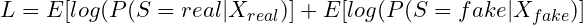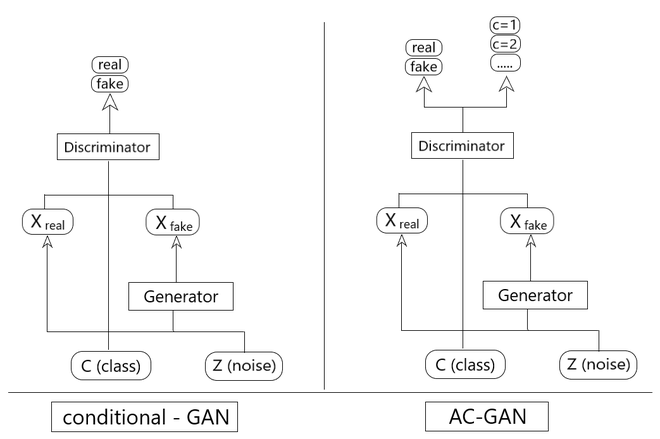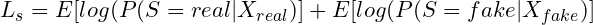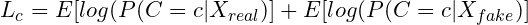# Understanding Auxiliary Classifier : GAN

• Last Updated : 14 Oct, 2020

In this article, we will be discussing a special class conditional GAN or c-GAN known as Auxiliary Classifier GAN or AC-GAN. Before getting into that, it is important to understand what a class conditional GAN is.

Attention reader! Don’t stop learning now. Get hold of all the important Machine Learning Concepts with the Machine Learning Foundation Course at a student-friendly price and become industry ready.

Class-Conditional GAN (c-GANs):

c-GAN can be understood as a GAN with some conditional parameters. We consider the original GAN framework and add prior information in the form of a class label. In c-GAN, an additional parameter ‘y’ is added to the Generator for generating the corresponding data. Labels are also put into the input to the Discriminator in order for the Discriminator to help distinguish the real data from the fake generated data.where,

L = Likelihood of predicting correctly.
S = Source (Real/Fake)
X = Input image
P = Probability DistributionTraining c-GAN

Intuition:

Looking at it intuitively, the Generator and Discriminator are two neural networks. The objective is to make the Generator generate images that are similar to the input images.

```Step 1 - The Generator (G) takes in the input image (x) and generates its own image of a shoe (G(x)).

Step 2 - The generated image and the input image is then fed to the Discriminator (D) that compares the two images
and tells if it is a real or a generated fake image.

Step 3 - So the Discriminator backpropagates information back to the Generator and tells it what was missing from
the generated image when compared to the original image (y).

Step 4 - Repeat the above three steps until the error generated by the Discriminator has reached the minimum.

This information makes the Generator generate more accurate images. This is how the c-GAN model is trained.
```c-GAN vs AC-GAN

Auxiliary Classifier: GANs

The auxiliary classifier GAN is simply an extension of class-conditional GAN that requires that the discriminator to not only predict if the image is ‘real’ or ‘fake’ but also has to provide the ‘source’ or the ‘class label’ of the given image.

For example, if the Generator generates the image of a shoe, the model has to predict if its a real image or fake as well as predict the ‘class labels’ of the real and generated images.

AC-GAN Architecture:

The AC-GAN architecture comprises generator 2 models :

Generator: It takes random points from a latent space as input and generates images.
Discriminator: It classifies images as either real (from the dataset) or fake (generated) as well as predict the class label.

In AC-GAN, the training of the basic GAN model has been improved.

Here, the generator is provided with two parameters instead of one. It gets random points from the latent space as well as a class label as input using which it attempts to generate an image for that class. The addition of the class label as input makes the image generation and classification process, dependent on the class label, hence the name. Using this Generator model, the training process becomes more stable and it can now be used to generate images of a specific type, using the class label.

The discriminator here is provided with both an image and the class label. So now, it has to classify whether the image is real or fake (same as before) and it also has to predict the class label of the image.

Here the objective function now has two parts:where,

LS = The likelihood of predicting the correct source.
S = Source
X = Input imagewhere

LS = The likelihood of predicting the correct class.
c = class label
X = Input image

The main objective for training the models is such that

• Discriminator is trained to maximize LC +LS.
• Generator is trained to maximize LC − LS.

Similar to the working of GAN model, a ‘minimax game’ takes place here, where the Discriminator is trying to maximize its reward (Lc + Ls) and the Generator is trying to minimize the Discriminator’s reward (Lc – Ls), i.e. maximize its loss.

The additional information provided, aids in better training of the model and generates much better output compared to the previous model.

Comparing efficiency with previous models:

In the earlier models, it was observed that increasing the number of classes while using the same model decreased the quality of the outputs generated by the model. But here, the AC-GAN model allows separation of large datasets into subsets (class-wise) and training the generator and discriminator models for each subset individually.

Structurally, this model is very similar to the existing GAN models. However, the changes done above to the base GAN model tends to give excellent results as well as stabilize the training process.

My Personal Notes arrow_drop_up